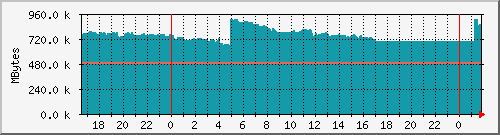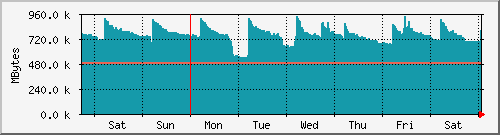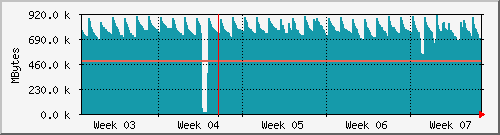# \$B%a%b%j6u\$-MFNL(B

\$B:G=*99?7F|;~(B 1:50, 2014\$BG/(B2\$B7n(B23\$BF|(B(\$BF|(B),
'www1'\$B\$N2TF/;~4V(B 0:24:14.
\$B0lF|%0%i%U(B(5\$BJ,4V(B\$BJ?6Q(B)\$B:GBgCM(B \$B 920.8 kMBytes (0.1%) \$BJ?6QCM(B \$B 759.6 kMBytes (0.1%) \$B8=:_CM(B \$B 867.7 kMBytes (0.1%) \$B:GBgCM(B \$B%9%o%C%W(B: 489.9 kMBytes (0.0%) \$BJ?6QCM(B \$B%9%o%C%W(B: 489.9 kMBytes (0.0%) \$B8=:_CM(B \$B%9%o%C%W(B: 489.9 kMBytes (0.0%)

\$B=54V%0%i%U(B(30\$BJ,4V(B\$BJ?6Q(B)\$B:GBgCM(B \$B 942.7 kMBytes (0.1%) \$BJ?6QCM(B \$B 768.4 kMBytes (0.1%) \$B8=:_CM(B \$B 811.6 kMBytes (0.1%) \$B:GBgCM(B \$B%9%o%C%W(B: 489.9 kMBytes (0.0%) \$BJ?6QCM(B \$B%9%o%C%W(B: 489.9 kMBytes (0.0%) \$B8=:_CM(B \$B%9%o%C%W(B: 489.9 kMBytes (0.0%)

\$B7n4V%0%i%U(B(2\$B;~4V(B\$BJ?6Q(B)\$B:GBgCM(B \$B 918.5 kMBytes (0.1%) \$BJ?6QCM(B \$B 771.4 kMBytes (0.1%) \$B8=:_CM(B \$B 704.6 kMBytes (0.1%) \$B:GBgCM(B \$B%9%o%C%W(B: 489.9 kMBytes (0.0%) \$BJ?6QCM(B \$B%9%o%C%W(B: 489.9 kMBytes (0.0%) \$B8=:_CM(B \$B%9%o%C%W(B: 489.9 kMBytes (0.0%)

\$BG/4V%0%i%U(B(1\$BF|(B\$BJ?6Q(B)\$B:GBgCM(B \$B 821.1 kMBytes (0.1%) \$BJ?6QCM(B \$B 779.3 kMBytes (0.1%) \$B8=:_CM(B \$B 792.1 kMBytes (0.1%) \$B:GBgCM(B \$B%9%o%C%W(B: 489.9 kMBytes (0.0%) \$BJ?6QCM(B \$B%9%o%C%W(B: 489.9 kMBytes (0.0%) \$B8=:_CM(B \$B%9%o%C%W(B: 489.9 kMBytes (0.0%)

 CYAN ### \$B RED ### \$B%9%o%C%W(B

 version 2.9.17 Tobias Oetiker and   Dave Rand

\$BF|K\8lLu(B(ISO-2022-JP)\$B:n@.(B \$B?">>(B \$BJ8FA(B <uematsu@kgz.com>